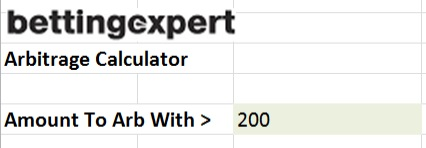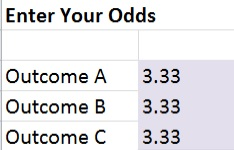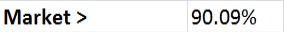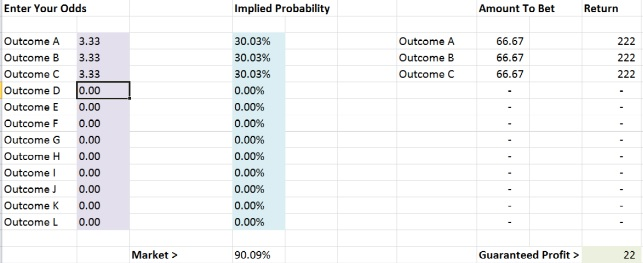This site contains commercial content# What Is Arbitrage Betting?

What is arbitrage betting? Here we show you how to calculate arbitrage bets plus our arbitrage excel spreadsheet available to download.

## What Is Arbitrage Betting?

Let’s take a look at two arbitrage betting examples. Example one, we encounter the following scenario:

### Balanced Odds

Andy Murray is playing Novak Djokovic in a Wimbledon Final.

• Murray is available at odds of 2.10 with bookmaker A
• Djokovic is likewise available at odds of 2.10 with bookmaker B

Here we find a perfect arbitrage opportunity:

Let’s say that Djokovic wins. Our returns would be:

• Murray -£100
• Djokovic +£110
• Profit = £10

### Unbalanced Odds

The above example was simple. But arbitrage betting opportunities are not always easy to identify. In the example below, the Chicago Bulls are facing the Cleveland Cavs in a NBA game:

• Cleveland are at odds of 1.20 with bookmaker A
• Chicago are at odds of 8.00 with bookmaker B.

This situation is a bit trickier. How can we calculate an arbitrage bet?

## How To Calculate An Arbitrage Bet

Calculating an arb with an arbitrage betting calculator is easy. But let’s look at the calculations involved to help us develop a deeper understanding of the dynamics involved in arbitrage betting.

There are two key questions when it comes to arbitrage betting:

1. How do you know if you have identified an arbitrage opportunity?
2. How much should you stake?

### Finding An Arbitrage Bet Opportunity

Similar to calculating the bookmaker’s margin (or commission) on a given betting market, we identify an arbitrage opportunity by adding the implied probabilities of the best betting odds available for each outcome.

To calculate the implied probability for each outcome, we simply divide the odds for that outcome in decimal format by 1.

So let’s then look at our simple example of Murray vs Djokovic from earlier in this article.

• Murray at odds of 2.10

implied probability = 1 / 2.10

= 0,476 = 47.6%

So the implied probability of Murray’s odds of 2.10 is 47.6%.

Let’s now consider Djokovic.

• Djokovic at odds of 2.10

implied probability = 1 / 2.10

= 0.476 = 47.6%

To determine if we have identified an arbitrage betting opportunity, we simply add the two implied probabilities together.

Total: 47.6% + 47.6% = 95.2%

When the implied probability for a given betting market adds up to less than 100%, it is an opportunity for an arbitrage bet. The lower the value below 100%, the better the arbitrage opportunity. In this example the market is well below 100%.

So lets then take a look at Cleveland vs Chicago in our NBA example. Have we identified an opportunity to arb?

• Cleveland at odds of 1.20

implied probability = 1 / 1.20

= 0.833 = 83.3%

• Chicago at odds of 8.00

implied probability = 1 / 8.00

= 0.125 = 12.5%

• Market Total

= 83.3% + 12.5% = 95.8%

Again, we have identified an arbitrage opportunity.

## How To Calculate Arbitrage Stakes

This question was easy to answer in our Murray vs Djokovic example. As both have the same odds, we simply bet the same amount on each outcome.

But more often than not, arbitrage bets are not this simplistic. Typically we will need to weigh our staking according to the odds of each outcome. How do we do this?

1. Calculate the implied probabilities of all possible market outcomes
2. Calculate the sum of the probabilities of all possible market outcomes
3. Divide the implied probability of each individual outcome by the sum of all probabilities

In this way we can determine the correct amount to bet.

Example: Cleveland vs Chicago

We calculate the implied probability of each outcome and add them together just as we previously did.

• Cleveland at odds of 1.20

implied probability = 1 / 1.20

= 0.833 = 83.3%

• Chicago at odds of 8.00

implied probability = 1 / 8.00

= 0.125 = 12.5%

• Market Total

= 83.3% + 12.5% = 95.8%

Now we calculate how much to bet on each outcome by dividing the implied probability of each outcome by the total market probability.

• Cleveland

= 83.3% / 95.8%

= 86.952%

• Chicago

= 12.5% / 95.8%

= 13.048%

So in this example, if we wanted to arb a total of £100, we would place 86.952% of our £100 on Cleveland at odds of 1.20 and 13.048% of our £100 on Chicago at odds of 8.00. This will see the same amount returned regardless of who wins the game, Cleveland or Chicago.

Let’s walk it through:

We want to stake £100 in our arbitrage bet.

£100 * 86.95% = £86.95 on Cleveland at odds of 1.20

£100 * 13.05% = £13.05 on Chicago at odds of 8.00

So now, here are the possibilities:

• If Cleveland wins, we collect (£86.95 * 1.20 =) £104.4. After we deduct our £100 stake, we come away with £4.40.
• If Chicago wins, we collect (£13.05 * 8.00) = £104.4. After we deduct our £100 stake, we come away with £4.40.

## Applying Our Calculation To Other Markets

We have only considered betting markets with just two outcomes, team A wins or team B wins. But what about a football 1X2 market or even a horse race featuring 12 runners? Well the principles remain the same.

1. Calculate the implied probabilities of all possible market outcomes
2. Calculate the sum of the probabilities of all possible market outcomes
3. Divide the implied probability of each individual outcome by the sum of all probabilities.

Let’s say we have an upcoming Premier League match between Manchester City and Arsenal. We are looking here at the 1X2 betting market. We have three possible outcomes:

• Manchester City win, at odds of 2.80
• Drawn match, at odds of 3.60
• Arsenal win, at odds of 3.20

Let’s see if we have an arbitrage opportunity. As we did in our previous examples, we calculate the implied probability for each individual outcome.

• Manchester City at odds of 2.80

implied probability = 1 / 2.80

= 0.3571 = 35.71%

• Drawn match at odds of 3.60

implied probability = 1 / 3.60

= 0.2778 = 27.78%

• Arsenal win at odds of 3.20

implied probability = 1 / 3.20

= 0.3125 = 31.25%

We then add each probability to come up with our market total.

= 35.71% + 27.78% + 31.25%

= 94.74%

Let’s say we want to stake a total of £100.

• Manchester City at odds of 2.80

= 35.71% / 94.74%

= 37.693%

So we will stake 37.693% of £100, a total of £37.69 on Manchester City to win.

• Drawn match at odds of 3.60

= 27.78% / 94.74%

= 29.323%

So we will stake 29.323% of £100, a total of £29.32 on the Drawn match.

• Arsenal at odds of 3.20

= 31.25% / 94.74%

= 32.984%

So we will stake 32.984% of £100, a total of £32.98 on Arsenal to win.

If we bet each of these amounts, we might expect positive results no matter what happens.

## Arbitrage Excel Arbitrage Calculator

We’ve made calculating arbitrage bets all the easier with our arbitrage excel calculator.

Here’s how:

Step 1 – Choose your preferred odds format by clicking on one of the tabs at the bottom of the sheet. Sheet 1 calculates decimal odds, sheet 2 calculates fractional odds and sheet 3 calculates American odds (also known as ‘Moneyline’ odds).

Step 2 – In the Amount To Arb With cell, enter the amount of money that you wish to invest in your “Arb”.Step 3 – Enter the best odds available for each possible outcome into Outcome cells. The bettingexpert Arbitrage Calculator allows you to enter odds for events with up to 10 outcomes.Step 4 – Have you identified an arbitrage opportunity? The percentage figure in the Market cell tells you.Step 5 – Once you have done so, the Amount To Bet column will tell you the exact amount to bet, while the other cell will tell you exact what the return will be.## Practical Problems In Arbitrage Betting

### 1. Limited Opportunity In Limited Time

In the Internet age, in most cases, it only takes up to 15 minutes until an arbitrage opportunity disappears. Spatial distance has no meaning on the Internet.

### 2. High turnover, low returns

Another issue is the diminutive size of typical returns. In almost every arbitrage betting opportunity you will return a relatively small profit from each transaction, a return of more than 3% on your investment will be a rare event.

### 3. Be careful with high stakes

Even if you have a large amount of money to invest, another problem soon arises. Few bookmakers are as transparent as Pinnacle are regarding their bet limits. Almost all other bookmakers accept large bets only when they are checked and waved through by a manager. So there is a real risk that one of your bets will not be accepted.

Chat